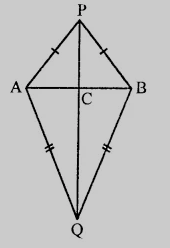"
">

# $AB$ is a line segment $P$ and $Q$ are points on opposite sides of $AB$ such that each of them is equidistant from the points $A$ and $B$. Show that the line $PQ$ is perpendicular bisector of $AB$."

Given:

$AB$ is a line segment $P$ and $Q$ are points on opposite sides of $AB$ such that each of them is equidistant from the points $A$ and $B$.

To do:

We have to show that the line $PQ$ is perpendicular bisector of $AB$.

Solution:

In $\triangle PAQ$ and $\triangle PBQ$,

$PA = PB$               (Given)

$QA = QB$               (Given)

$PQ = PQ$               (Common)

Therefore, by SSS axiom,

$\triangle PAQ \cong \triangle PBQ$

This implies,

$\angle APQ = \angle BPQ$               (CPCT)

In $\triangle APC$ and $\triangle BPC$,

$PA = PB$         (Given)

$\angle APC = \triangle BPC$

$PC = PC$             (Common)

Therefore, by SAS axiom,

$\triangle APC \cong \triangle BPC$

This implies,

$AC = BC$                (CPCT)

$\angle PCA = \angle PCB$           (CPCT)

$\angle PCA + \angle PCB = 180^o$           (Linear pair)

This implies,

$\angle PCA = \angle PCB = 90^o$

Therefore, $PQ$ is the perpendicular bisector of $AB$.

Hence proved.

Updated on: 10-Oct-2022

29 Views